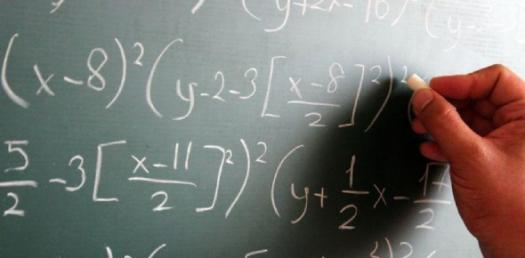# Dimensional Analysis Questions! Math Quiz

10 Questions | Attempts: 4439
ShareSettingsDimensional analysis questions math quiz. This analysis is based on checking the relationships between different physical qualities. There are prescribed instructions that one should follow when undertaking this analysis. If you want to test out how much you understand it, this quiz is for you to refresh your understanding. Do give it a shot and see how well you will do answering the analysis questions below. All the best as you tackle them.

• 1.
Which of the following is NOT an SI unit of measure?
• A.

Kilogram

• B.

Meter

• C.

Foot

• D.

Second

• 2.
Which of the following prefixes will multiply the unit by 1,000?
• A.

Kilo-

• B.

Mega-

• C.

Centi-

• D.

Milli-

• 3.
Which of the following are English units of measure?
• A.

Foot

• B.

Pound

• C.

Second

• D.

All of the above

• 4.
The liter is an International System (SI) measure of:
• A.

Time

• B.

Volume

• C.

Length

• D.

Mass

• 5.
You are given an object weighing 10 kilograms.  What is the mass in English units?
• A.

2.2 pounds

• B.

22 pounds

• C.

0.22 pounds

• D.

None of the above

• 6.
Your coach tells the team that practice will include a 5-kilometer "fun" run.  How many miles will you be running for "fun?"
• A.

1.6 miles

• B.

0.31 miles

• C.

3.1 miles

• D.

16 miles

• 7.
While traveling in Canada, a police officer pulls you over for speeding.  The posted speed limit is 100 kilometers per hour.  She asks you if you know how fast you were driving.  You suddenly realize that your speedometer's "100" measures miles per hour, not kilometers per hour.  How fast were you going?
• A.

100 kilometers per hour

• B.

62 kilometers per hour

• C.

126 kilometers per hour

• D.

161 kilometers per hour

• 8.
Your friend wants to know his height using the SI system.  He is six feet tall; what is the equivalent height in meters?
• A.

1.83 meters

• B.

6 meters

• C.

0.83 meters

• D.

1.38 meters

• 9.
How many centimeters are there in one inch?
• A.

25.44

• B.

0.39

• C.

2.54

• D.

1

• 10.
When measuring time, a second in the SI system is the same as a second in the English system.
• A.

True

• B.

False

## Related TopicsBack to top
×

Wait!
Here's an interesting quiz for you.